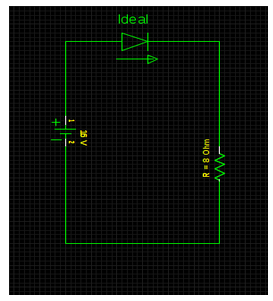# Compute the current in an ideal diode and power consumed by 8 ohm resistor of the below drawn circuit.

## Compute the current in (a) an ideal diode and (b) power consumed by 8 ohm resistor of the below drawn circuit.

Option:a. a. 2 A & 24 W
b. 2A & 32 W
c. 2A & 12 W
d. 2A & 8 W

Correct Answer: b. 2A & 32 W

Explanation:

Above schematic shows an ideal diode operating in forward biased direction. Therefore, (a) current in the circuit can be estimated by,
I = V/ R = 16 / 8 = 2 A
In forward direction, current flows but there is no voltage drop. Thus, the power dissipated by an ideal diode is zero.

On the contrary, in reverse direction, voltage exists but there is lack of current. Hence, power dissipated by an ideal diode again becomes zero for this condition.

However, it is obvious that an ideal diode never dissipates any power.

(b) Power consumed by 8 ohm resistor = I2 R = (2)2 x 8 = 32 W